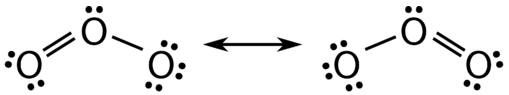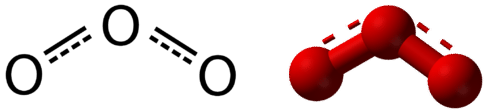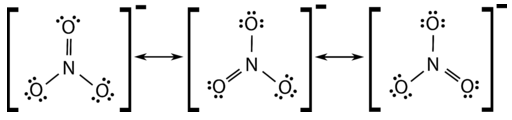# 5.5: Resonance - Equivalent Lewis Structures for the Same Molecule

$$\newcommand{\vecs}{\overset { \rightharpoonup} {\mathbf{#1}} }$$ $$\newcommand{\vecd}{\overset{-\!-\!\rightharpoonup}{\vphantom{a}\smash {#1}}}$$$$\newcommand{\id}{\mathrm{id}}$$ $$\newcommand{\Span}{\mathrm{span}}$$ $$\newcommand{\kernel}{\mathrm{null}\,}$$ $$\newcommand{\range}{\mathrm{range}\,}$$ $$\newcommand{\RealPart}{\mathrm{Re}}$$ $$\newcommand{\ImaginaryPart}{\mathrm{Im}}$$ $$\newcommand{\Argument}{\mathrm{Arg}}$$ $$\newcommand{\norm}{\| #1 \|}$$ $$\newcommand{\inner}{\langle #1, #2 \rangle}$$ $$\newcommand{\Span}{\mathrm{span}}$$ $$\newcommand{\id}{\mathrm{id}}$$ $$\newcommand{\Span}{\mathrm{span}}$$ $$\newcommand{\kernel}{\mathrm{null}\,}$$ $$\newcommand{\range}{\mathrm{range}\,}$$ $$\newcommand{\RealPart}{\mathrm{Re}}$$ $$\newcommand{\ImaginaryPart}{\mathrm{Im}}$$ $$\newcommand{\Argument}{\mathrm{Arg}}$$ $$\newcommand{\norm}{\| #1 \|}$$ $$\newcommand{\inner}{\langle #1, #2 \rangle}$$ $$\newcommand{\Span}{\mathrm{span}}$$$$\newcommand{\AA}{\unicode[.8,0]{x212B}}$$

##### Learning Objectives
• Explain the concept of resonance and how it works with within molecules.

## Resonance

There are some cases in which more than one viable Lewis structure can be drawn for a molecule. An example is the ozone $$\left( \ce{O_3} \right)$$ molecule in Figure $$\PageIndex{1}$$. There are a total of 18 electrons in the structure and so the following two structures are possible.Figure $$\PageIndex{1}$$: Resonance forms of ozone. Note the use of the double-headed arrow.

The structure on the left ($$\PageIndex{1}$$) can be converted to the structure on the right by a shifting of electrons without altering the positions of the atoms.

It was once thought that the structure of a molecule such as $$\ce{O_3}$$ consisted of one single bond and one double bond which then shifted back and forth as shown above. However, further studies showed that the two bonds are identical. Any double covalent bond between two given atoms is typically shorter than a single covalent bond. Studies of the $$\ce{O_3}$$ and other similar molecules showed that the bonds were identical in length. Interestingly, the length of the bond is in between the lengths expected for an $$\ce{O-O}$$ single bond and a double bond.

Resonance is the use of two or more Lewis structures to represent the covalent bonding in a molecule. One of the valid structures is referred to as a resonance structure. It is now understood that the true structure of a molecule which displays resonance is that of an average or a hybrid of all the resonance structures. In the case of the $$\ce{O_3}$$ molecule, each of the covalent bonds between $$\ce{O}$$ atoms are best thought of as being "one and a half" bonds, as opposed to either a pure single bond or a pure double bond. This "half-bond" can be shown as a dotted line in both the Lewis structure and the molecular model (Figure $$\PageIndex{2}$$).Figure $$\PageIndex{2}$$: "Half-bond" model of ozone molecule. This is a better description of the electronic structure of ozone than either of the resonance structures in Figure $$\PageIndex{1}$$.

Many polyatomic ions also display resonance. In some cases, the true structure may be an average of three valid resonance structures, as in the case of the nitrate ion, $$\ce{NO_3^-}$$ in Figure $$\PageIndex{3}$$.Figure $$\PageIndex{3}$$: Resonance structure of nitrate anion.

The bond lengths between the central $$\ce{N}$$ atom and each $$\ce{O}$$ atom are identical and the bonds can be approximated as being equal to one and one-third bonds.

## Summary

• Resonance structures are averages of different Lewis structure possibilities.
• Bond lengths are intermediate between covalent bonds and covalent double bonds.

5.5: Resonance - Equivalent Lewis Structures for the Same Molecule is shared under a not declared license and was authored, remixed, and/or curated by LibreTexts.